Need a discount on popular programming courses? Find them here. View offers

Don't have an account?

Have you read our submission guidelines?

Go back to

# Decision Tree in Machine Learning

## What is a Decision Tree?

Decision Tree using a tree-like structure, as a predictive model, to explicitly represent the decision and decision making. Each internal node of the Decision Tree is a feature and each outgoing edge from that node represents the value that feature can take.

In the case of a categorical feature, the number of outgoing edges is the number of different values in those categories. In the case of a numerical feature, the number of outgoing edges is generally two, one in which the feature value is less than a real-valued number and other which is greater. Each leaf node represents a class label. The feature at each node is chosen based on the Information Gain and the one with the maximum gain is more important and is chosen at a higher level(closer to the root node).

## Building a Decision Tree

We shall learn how to build a decision tree with the help of an example.

In this example, a dataset defines the attributes of the superheroes, and based on the training the model then evaluates whether your date is a good or bad superhero. Consider the following dataset.

 Superhero mask cape tie ears smokes height class Batman y y n y n 180 good Robin y y n n n 176 good Alfred n n y n n 185 good Penguin n n y n y 140 evil Catwoman y n n y n 170 evil Joker n n n n n 179 evil Batgirl y y n y n 165 ? Riddler y n n n n 182 ? Your date n y y y y 181 ?

One possible Decision Tree covering all the aspects and test cases would be: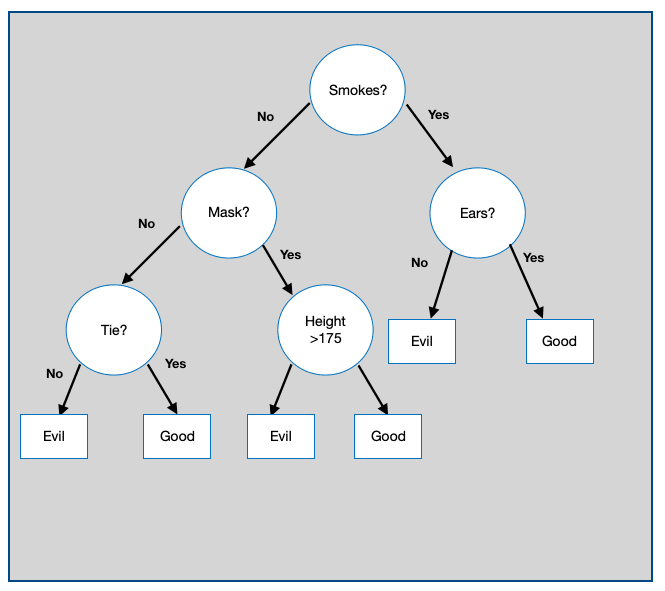Building and splitting the tree and making it complicated is not recommended as although it gives zero percent testing error but it leads overfitting. So, the aim is to find the smallest tree that gets the trained data set alright.

Let us now see the pseudo-code for building an efficient decision tree.

The pseudo-code is as follows:

### procedure BUILDSUBTREE (SET OF TRAINING INSTANCES D)

1. C <- Determine the candidate splits(D)
2. if stopping criteria met then
3. make a leaf node N

1. Determine the class label for N

### else

1. make an internal node N
2. S <- Find the best split(C,D)
3. for each outcome o of S
4. Do <- subset of instances that have outcome o
5. oth child of N <- BuildSubTree(Do)
6. return the subtree rooted at N

So following this the smallest tree possible is: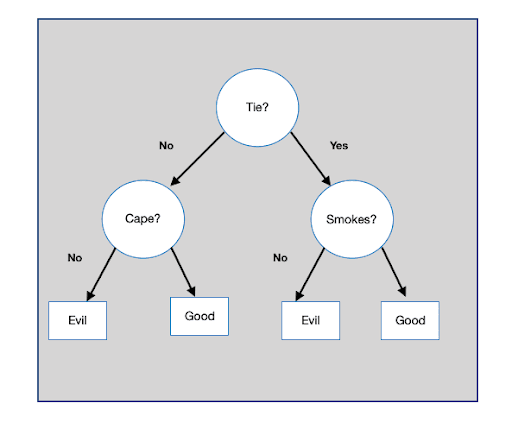Decision Tree is not an efficient algorithm, however, they have clear bias-variance problems that become easy to address those problems. Variance can be adjusted with bagging and bias with boosting.

## Entropy

Entropy is the measure of uncertainty associated with a random variable, Y. It is the expected number of bits required to communicate the value of the variable.

`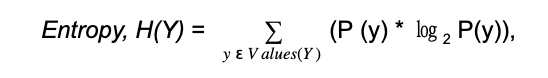`

where P(y) is the probability of Y having the value y. With respect to Decision Trees, the entropy is used to find the best feature split at any node.

## Splitting Rules Used By Different Decision Tree Algorithms

### 1. Information Gain

Information Gain is the change in the Entropy, H from a prior state to a new state when splitting on a feature:

``

Information gain is used to identify the best feature to split the given training dataset. It selects the split S that most reduces the conditional entropy of the output Y of the training dataset D.

Information Gain is calculated for all the features and the feature with the highest gain is chosen as the most important feature.

### 2. Gain Ratio

Gain Ratio normalizes the information gain dividing it by entropy of the split being considered, thereby avoiding the unjustified favoritism of Information Gain.

``

### 3. Permutation Test

The labels of the organic data are permuted and the statistic to be tested is calculated for the relabeled data for all the possible permutations of the labels. The test statistics value for the original data compared with values obtained over all of the permutations, by calculating the percentage of the latter that is at least as extreme as the former.

Suggested Course

### 4. Multivariate Split

Multivariate decision trees can use split that contain more than one attribute at each internal node.

### 5. Impurity Function and Gini Index

Impurity Function: Functions that measure how pure the label is.

Gini Impurity: For a set of data points S,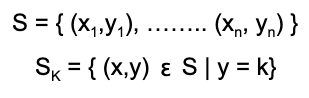Probability of picking a point with a certain label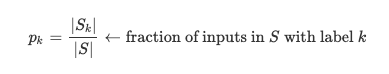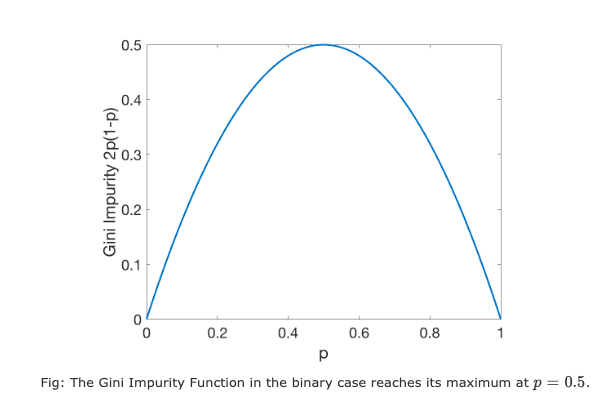Gini Index: It is a measure of how often a randomly chosen instance from the dataset would be incorrectly labeled if it was labeled randomly according to the distribution of labels in the subset.

## Pruning

Pruning is a technique that reduces the complexity of the final classifier by removing the subtrees from it whose existence does not impact the accuracy of the model. In pruning, you grow the complete tree and then iteratively prune back some nodes until further pruning is harmful. This is done by evaluating the impact of pruning each node on the tuning (validation) dataset accuracy and greedily removing the one that most improves the data accuracy.

Decision Tree can be pruned by imposing a minimum on the number of training examples that reach a leaf. Pruning keeps the trees simple without affecting the overall accuracy. It helps solve the overfitting issue by reducing the size as well as the complexity of the tree.

### Code

`def main(name):for i in range(len(date_data)):superhero_data = dict(zip(header,date_data[i]))if superhero_data["Superhero"].lower()==name.lower():breakreturn smoke(superhero_data)def smoke(superhero_data):"To check whether the Superhero Smoke or Not"if superhero_data["Smoker"]=="y":return ears(superhero_data)else:return mask(superhero_data)def ears(superhero_data):if superhero_data["Ears"]=="y":return "Good"else:return "Evil"def mask(superhero_data):if superhero_data['Mask']=="y":return height(superhero_data)else:return tie(superhero_data)def height(superhero_data):if superhero_data["Height"]>175:return"Good"else:return "Evil"def tie(superhero_data):if superhero_data["Tie"]=="y":return "Good"else:return "Evil"if __name__=="__main__":date_data = [ ["Batman","y","y","n","y","n",180],["Robin","y","y","n","n","n",176],["Alfred","n","n","y","n","n",185],["Penguin","n","n","y","n","y",140],["Catwomen","y","n","n","y","n",170],["Joker","n","n","n","n","n",179],["Batgirl","y","y","n","y","n",165],["Riddler","y","n","n","n","n",182],["Your Date","n","y","y","y","y",181],]header=["Superhero", "Mask", "Cape", "Tie", "Ears", "Smoker", "Height", "Class"]print("-----Is your date a good or bad psychopath---- ")for i in range(len(date_data)):print(date_data[i],"is --->",end="")print(main(date_data[i]))`

Output:

`-----Is your date a good or bad psychopath----Batman is --->GoodRobin is --->GoodAlfred is --->GoodPenguin is --->EvilCatwoman is --->EvilJoker is --->EvilBatgirl is --->EvilRiddler is --->GoodYour Date is --->Good`

## Why Use A Decision Tree? Advantages of a Decision Tree

When we fit a Decision Tree to a training dataset, the top few nodes on which the tree is split are basically the most important features in the dataset and thus, you can use it as the feature selection technique to select the most relevant features in the dataset. Decision trees are also insensitive to outliers since the splitting happens based on the proportion of samples within the split ranges and not so absolute values.

Because of their tree-like structure, they are very easy to understand and interpret. They do not need the data to be normalized and work well even when the features have a nonlinear relation with each other.

### Disadvantages of using a Decision Tree Algorithm

1. Even a small change in input data can, at times, cause large changes in the tree as it may drastically impact the information gain used by Decision Trees to select the features.
2. Decision Trees moreover, examine only a single field at a time, leading to rectangular classification boxes. This may not correspond well with the actual distribution of records in the decision space.
3. Decision Trees are inadequate when it comes to applying regression and predicting continuous values. A continuous variable can have an infinite number of values within an interval, capturing which, in a tree having only a finite number of branches and leaves, is very hard.
4. There is a possibility of duplication with the same subtree on different paths, leading to complex trees.
5. Every feature in the tree is forced to interact with every feature further up the tree. This is extremely inefficient if there are features that have no or weak interactions.

## Conclusion

Decision Tree is an NP-hard problem i.e. if the dataset gets larger the amount of computation time to be done to accommodate the extra data grows exponentially. Its aim is to find the smallest tree that trains the system correctly. We hope you understood this machine learning concept. You may be interested in learning about more Machine Learning Algorithms here.

Which is your favourite machine learning concept? Let us in the comments below!

STAY IN LOOP TO BE AT THE TOP

#### Welcome to the club and Thank you for subscribing!

By Simran Kaur Arora

Simran works at Hackr as a technical writer. The graduate in MS Computer Science from the well known CS hub, aka Silicon Valley, is also an editor of the website. She enjoys writing about any tech topic, including programming, algorithms, cloud, data science, and AI. Traveling, sketching, and gardening are the hobbies that interest her.

View all post by the author

Disclosure: Hackr.io is supported by its audience. When you purchase through links on our site, we may earn an affiliate commission.